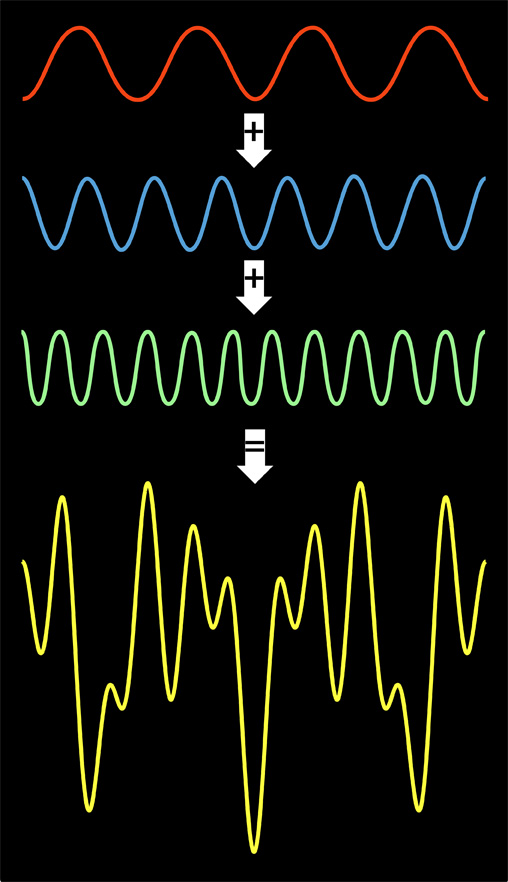Theory Evaluation Code Applications Hardware Publications News People Workshops## Sparse Fast Fourier Transform :

The discrete Fourier transform (DFT) is one of the most important and widely used computational tasks. Its applications are broad and include signal processing, communications, and audio/image/video compression. Hence, fast algorithms for DFT are highly valuable. Currently, the fastest such algorithm is the Fast Fourier Transform (FFT), which computes the DFT of an n-dimensional signal in O(nlogn) time. The existence of DFT algorithms faster than FFT is one of the central questions in the theory of algorithms.

A general algorithm for computing the exact DFT must take time at least proportional to its output size n. In many applications, however, most of the Fourier coefficients of a signal are small or equal to zero, i.e., the output of the DFT is sparse. This is the case for video signals, where a typical 8x8 block in a video frame has on average 7 non-negligible frequency coefficients (i.e., 89% of the coefficients are negligible). For sparse signals, the Ω(n) lower bound for the complexity of DFT no longer applies. If a signal has a small number k of non-zero Fourier coefficients the output of the Fourier transform can be represented succinctly using only k coefficients. Hence, for such signals, we can find DFT algorithms whose runtime is sublinear in the signal size, n.

We present here several new results for sparse Fourier transform:

- An O(k log n)-time algorithm for the exactly k-sparse case.

- An O(k log n log(n/k))-time algorithm for the general case.

- An Ω(k log(n/k) /loglog n) lower bound for sample complexity.

Both algorithms improve over FFT, for any k = o(n). Moreover, if one assume that FFT is optimal, the algorithm for the exactly k-sparse case is optimal. Under the same assumption, the result for the general case is at most one loglog n factor away from the optimal runtime for the case of “large” sparsity k = n/log n.

We also have several follow up results that improve the sample complexity of the algorithms. A summary of all the results can be found under the Theory section.

We further demonstrate that the sparse Fourier transfrom has impact on several practical applications in the areas of wireless communication, medical imaging, and computational photography. The details of these applications can be found under the Applications section.

This research is supported in part by NSF and DARPA.A Faster Fourier Transform
A mathematical upgrade promises a speedier digital world.
Mark Anderson, Technology ReviewThe Faster-than-Fast Fourier Transform
For a large range of practically useful cases, MIT researchers find a way to increase the speed of one of the most important algorithms in the information sciences.
Larry Hardesty, MIT News OfficeA Faster Fast Fourier Transform
New algorithm crunches sparse data with speed
David Schneider, IEEE Spectrum